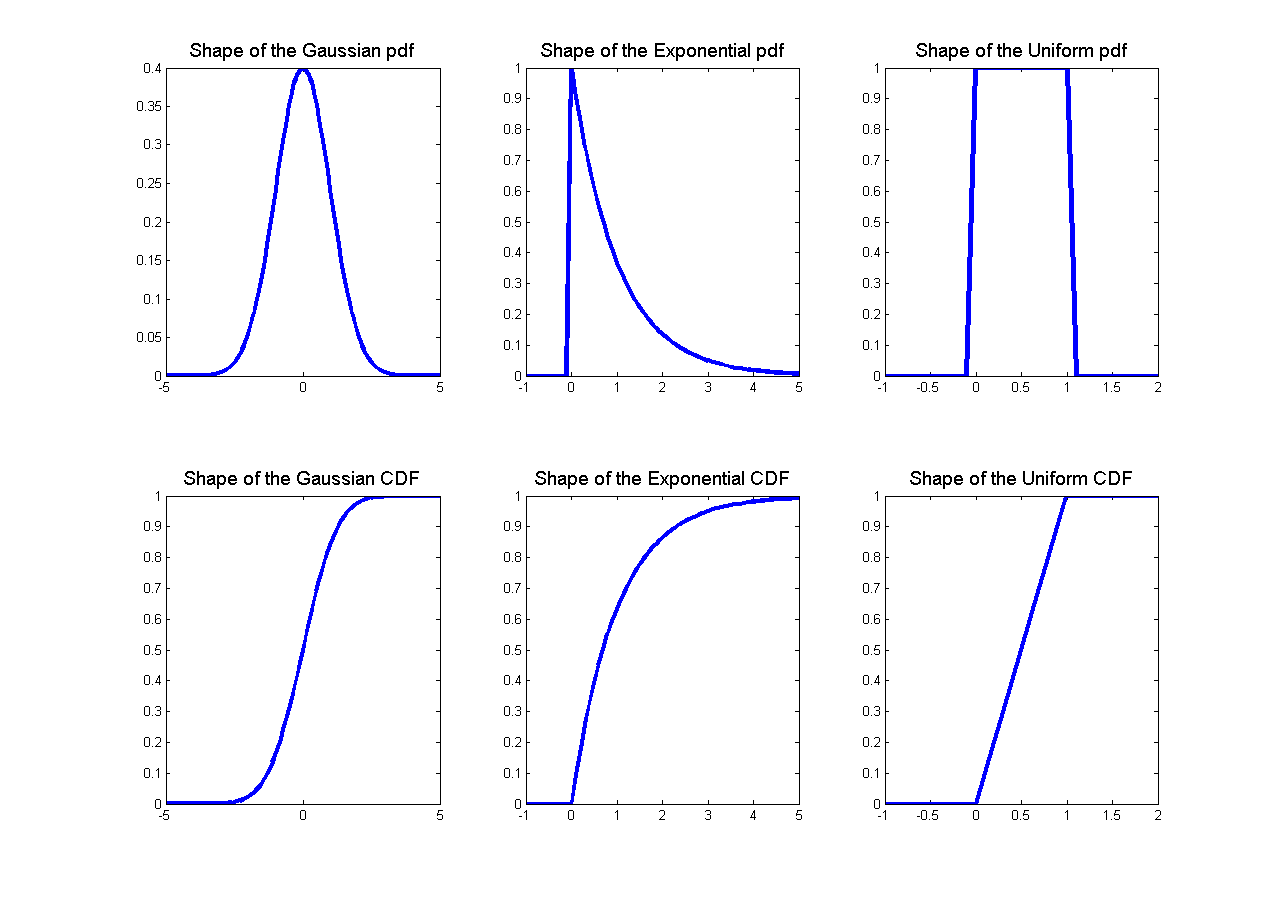# CS229 机器学习课程复习材料-概率论

## 概率论复习和参考

### 1. 概率的基本要素

• 样本空间$\Omega$：随机实验的所有结果的集合。在这里，每个结果 $w \in \Omega$ 可以被认为是实验结束时现实世界状态的完整描述。

• 事件集（事件空间）$\mathcal{F}$：元素 $A \in \mathcal{F}$ 的集合（称为事件）是 $\Omega$ 的子集（即每个 $A \subseteq \Omega$ 是一个实验可能结果的集合）。

备注：$\mathcal{F}$需要满足以下三个条件：

(1) $\emptyset \in \mathcal{F}$

(2) $A \in \mathcal{F} \Longrightarrow \Omega \backslash A \in \mathcal{F}$

(3) $A_1,A_2,\cdots A_{i} \in \mathcal{F}\Longrightarrow\cup_{i} A_{i} \in \mathcal{F}$

• 概率度量$P$：函数$P$是一个$\mathcal{F} \rightarrow \mathbb{R}$的映射，满足以下性质：

• 对于每个 $A \in \mathcal{F}$$P(A) \geq 0$,
• $P(\Omega) = 1$
• 如果$A_1 ,A_2 ,\cdots$ 是互不相交的事件 (即 当$i \neq j$时，$A_{i} \cap A_{j}=\emptyset$ ), 那么：

• 如果$A \subseteq B$，则：$P(A) \leq P(B)$
• $P(A \cap B) \leq min(P(A),P(B) )$
• (布尔不等式)：$P(A \cup B) \leq P(A)+P(B)$
• $P(\Omega |A ) =1-P(A)$
• (全概率定律)：如果$A_1，\cdots，A_k$是一些互不相交的事件并且它们的并集是$\Omega$，那么它们的概率之和是1

### 2. 随机变量

#### 2.1 累积分布函数• $0 \leq F_{X}(x)\leq 1$
• $\lim _{x \rightarrow-\infty} F_{X}(x)=0$
• $\lim _{x \rightarrow\infty} F_{X}(x)=1$
• $x \leq y \Longrightarrow F_{X}(x)\leq F_{X}(y)$

#### 2.2 概率质量函数

• $0 \leq p_{X}(x)\leq 1$
• $\sum_{x \in V \text { al }(X)} p_{X}(x)=1$
• $\sum_{x \in A} p_{X}(x)=P(X \in A)$

#### 2.3 概率密度函数

CDFPDF(当它们存在时！)都可用于计算不同事件的概率。但是应该强调的是，任意给定点的概率密度函数(PDF)的值不是该事件的概率，即$f _X (x) \not = P(X = x)$。例如，$f _X (x)$可以取大于1的值(但是$f _X (x)$$\mathbb{R}$的任何子集上的积分最多为1)。

• $f_X(x)\geq 0$
• $\int_{-\infty}^{\infty} f_{X}(x)=1$
• $\int_{x \in A} f_{X}(x) d x=P(X \in A)$

#### 2.4 期望

• 对于任意常数 $a \in \mathbb{R}$$E[a]=a$
• 对于任意常数 $a \in \mathbb{R}$$E[af(X)]=aE[f(X)]$
• (线性期望)：$E[f(X)+g(X)]=E[f(X)]+E[g(X)]$
• 对于一个离散随机变量$X$$E[1\{X=k\}]=P(X=k)$

#### 2.5 方差

• 对于任意常数 $a \in \mathbb{R}$$Val[a]=0$
• 对于任意常数 $a \in \mathbb{R}$$Var[af(X)]=a^2Var[f(X)]$

#### 2.6 一些常见的随机变量

• 伯努利分布：硬币掷出正面的概率为$p$（其中：$0 \leq p \leq 1$），如果正面发生，则为1，否则为0。

• 二项式分布：掷出正面概率为$p$（其中：$0 \leq p \leq 1$）的硬币$n$次独立投掷中正面的数量。

• 几何分布：掷出正面概率为$p$（其中：$p >0$）的硬币第一次掷出正面所需要的次数。

• 泊松分布：用于模拟罕见事件频率的非负整数的概率分布（其中：$\lambda >0$）。

• 均匀分布：在$a$$b$之间每个点概率密度相等的分布（其中：$a）。
• 指数分布：在非负实数上有衰减的概率密度（其中：$\lambda >0$）。
• 正态分布：又被称为高斯分布。### 3. 两个随机变量

#### 3.1 联合分布和边缘分布

• $0 \leq F_{XY }(x,y) \leq 1$
• $\lim _{x, y \rightarrow \infty} F_{X Y}(x, y)=1$
• $\lim _{x, y \rightarrow -\infty} F_{X Y}(x, y)=0$
• $F_{X}(x)=\lim _{y \rightarrow \infty} F_{X Y}(x, y)$